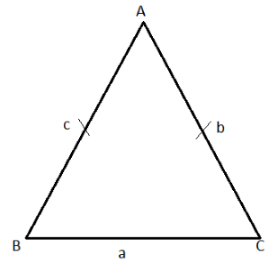The perimeter of an isosceles triangle is equal to 14cm, the lateral side is to the base in the ratio 5:4. The area of the triangle is[].

# The perimeter of an isosceles triangle is equal to 14cm, the lateral side is to the base in the ratio 5:4. The area of the triangle is[].

Fill Out the Form for Expert Academic Guidance!l

+91

Live ClassesBooksTest SeriesSelf Learning

Verify OTP Code (required)

### Solution:

Given:Lateral side of the triangle:Base side of the triangle:PerimeterThe perimeter of the triangle is equal to the sum of all the three sides of the triangle.
Thus,Simplify, we get,Semi-perimeter is half of the perimeter:Now, using the Heron’s formula:Simplify the above equation:Hence, the area of the triangle is:## Related content

 Area of Square Area of Isosceles Triangle Pythagoras Theorem Triangle Formula Perimeter of Triangle Formula Area Formulae Volume of Cone Formula Matrices and Determinants_mathematics Critical Points Solved Examples Type of relations_mathematics+91

Live ClassesBooksTest SeriesSelf Learning

Verify OTP Code (required)